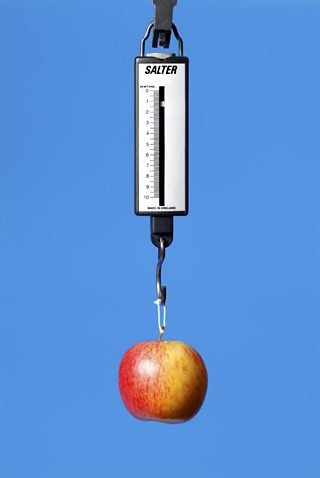# What are forces?

A can be a push or a pull. For example, when you push open a door you have to apply a force to the door. You also have to apply a force to pull open a drawer.

You cannot see a force but often you can see what it does. When a force is exerted on an object, it can change the object’s:

• speed
• direction of movement
• shape (for example, an elastic band gets longer if you pull it)A force meter, also called a newton meter, is used to measure forces

Forces can be contact forces, where objects must touch each other to exert a force. Other forces are non-contact forces, where objects do not have to touch each other. These include:

• gravity
• magnetism
• forces due to static electricity

## Measuring forces

Forces can be measured using a force meter, also called a newton meter. Force meters contain a spring connected to a metal hook. The spring stretches when a force is applied to the hook. The bigger the force applied, the longer the spring stretches and the bigger the reading.

The unit of force is called the , and it has the symbol N. The greater the force, the bigger the number, so 100 N is a greater force than 5 N.